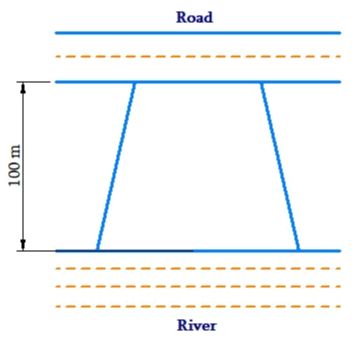# Ex.11.2 Q8 Mensuration Solution - NCERT Maths Class 8

Go back to  'Ex.11.2'

## Question

Mohan wants to buy a trapezium shaped field. Its side along the river is parallel to and twice the side along the road. If the area of this field is $$10500\;\rm{m^2}$$and the perpendicular distance between the two parallel sides is $$100\,\rm{m}$$, find the length of the side along the river.Video Solution
Mensuration
Ex 11.2 | Question 8

## Text Solution

What is Known?

The field is trapezium shaped. The area of this field and perpendicular distance between the two parallel sides are known.

What is unknown?

Length of the field side along the river.

Reasoning:

Since area of the trapezium is known. So is easy to find another parallel side by using given information.

Steps:

Let the length of the field along the road be $$l$$ meter

Hence the length of the of the field along the river will be $$2l$$ meter

Area of the trapezium=$$\frac{1}{2}\times$$ (Sum of parallel side)$$\times$$ (Distance between the parallel sides)
\begin{align}10500\,\,{{\text{m}}^{2}}&=\frac{1}{2}\times (l+2l)\times 100\,\,\text{m} \\10500\,\,{{\text{m}}^{2}}&=\frac{1}{2}\times 3l\times 100\,\,\text{m} \\10500\,{{\text{m}}^{2}}&=3l\times 50 \,\rm{m} \\150\times l&=10500\,\text{m} \\l&=70\,\,\text{m}\end{align}

Thus, length of the field along the river $$= 2 \times l = 2 \times 70\,\,{\rm{m}} = 140\,\,{\rm{m}}$$.

Learn from the best math teachers and top your exams

• Live one on one classroom and doubt clearing
• Practice worksheets in and after class for conceptual clarity
• Personalized curriculum to keep up with school